How Cheenta works to ensure student success?
Explore the Back-Story

# TIFR 2015 Problem 7 Solution -Increasing Function and ContinuityTIFR 2015 Problem 7 Solution is a part of TIFR entrance preparation series. The Tata Institute of Fundamental Research is India's premier institution for advanced research in Mathematics. The Institute runs a graduate program leading to the award of Ph.D., Integrated M.Sc.-Ph.D. as well as M.Sc. degree in certain subjects.
The image is a front cover of a book named Introduction to Real Analysis by R.G. Bartle, D.R. Sherbert. This book is very useful for the preparation of TIFR Entrance.

Also Visit: College Mathematics Program of Cheenta

## Problem:

Let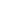and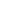be two functions from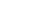towithstrictly increasing. Which of the following statements is always correct?

A. If (g) is continuous, thenis continuous

B. If  (f) is continuous, thenis continuous

C. If (f) andis continuous, then (g) is continuous

D. If (g) andare continuous, then (f) is continuous

## Discussion:

A: Let (g(x)=x) for all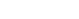.

(f(x)=x) for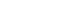and (f(x)=5+x) for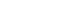.

Then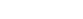and (f) is not continuous.

So A is False.

B: Reverse (f) and (g) in A to show that B is False.

C: If (f) andare continuous then (f) is 1-1 (increasing), continuous map ([0,1]to [0,1]).

(A subset [0,1] ) be closed. Then (A) is compact. (Closed subsets of compact spaces are compact).

Therefore (f(A)) is compact. (continuous image of compact set is compact).

We have that (f(A)) is a compact subset of ([0,1]). Therefore (f(A)) is closed in ([0,1]). (compact subspace of Hausdorff space is closed).

Therefore, (f) is a closed map. So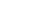is continuous.

Hence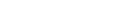is continuous.

So, C is True.

D: Let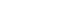for all.

(f(x)=x) forand (f(x)=5+x) for.

Then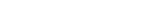for all.

Sois continuous but (f) is not continuous.

So, D is False.

## Helpdesk

• What is this topic: Real Analysis
• What are some of the associated concept: Continuity,Closed Set, Compact Set
• Book Suggestions: Introduction to Real Analysis by R.G. Bartle, D.R. SherbertTIFR 2015 Problem 7 Solution is a part of TIFR entrance preparation series. The Tata Institute of Fundamental Research is India's premier institution for advanced research in Mathematics. The Institute runs a graduate program leading to the award of Ph.D., Integrated M.Sc.-Ph.D. as well as M.Sc. degree in certain subjects.
The image is a front cover of a book named Introduction to Real Analysis by R.G. Bartle, D.R. Sherbert. This book is very useful for the preparation of TIFR Entrance.

Also Visit: College Mathematics Program of Cheenta

## Problem:

Letandbe two functions fromtowithstrictly increasing. Which of the following statements is always correct?

A. If (g) is continuous, thenis continuous

B. If  (f) is continuous, thenis continuous

C. If (f) andis continuous, then (g) is continuous

D. If (g) andare continuous, then (f) is continuous

## Discussion:

A: Let (g(x)=x) for all.

(f(x)=x) forand (f(x)=5+x) for.

Thenand (f) is not continuous.

So A is False.

B: Reverse (f) and (g) in A to show that B is False.

C: If (f) andare continuous then (f) is 1-1 (increasing), continuous map ([0,1]to [0,1]).

(A subset [0,1] ) be closed. Then (A) is compact. (Closed subsets of compact spaces are compact).

Therefore (f(A)) is compact. (continuous image of compact set is compact).

We have that (f(A)) is a compact subset of ([0,1]). Therefore (f(A)) is closed in ([0,1]). (compact subspace of Hausdorff space is closed).

Therefore, (f) is a closed map. Sois continuous.

Henceis continuous.

So, C is True.

D: Letfor all.

(f(x)=x) forand (f(x)=5+x) for.

Thenfor all.

Sois continuous but (f) is not continuous.

So, D is False.

## Helpdesk

• What is this topic: Real Analysis
• What are some of the associated concept: Continuity,Closed Set, Compact Set
• Book Suggestions: Introduction to Real Analysis by R.G. Bartle, D.R. Sherbert

This site uses Akismet to reduce spam. Learn how your comment data is processed.

### Knowledge Partner